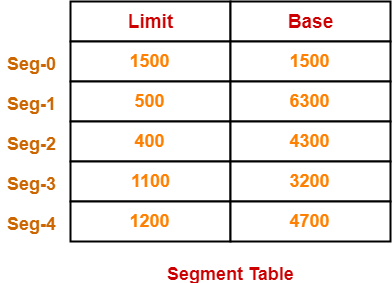# Segmentation in OS | Practice Problems

## Segmentation in OS-

Before you go through this article, make sure that you have gone through the previous article on Segmentation in OS.

We have discussed-

• Like Paging, Segmentation is another non-contiguous memory allocation technique.
• Segmentation divides the process into meaningful segments.
• Segment table stores the information about each segment of the process.

In this article, we will discuss a practice problem based on segmentation.

## Problem-

Consider the following segment table-

 Segment No. Base Length 0 1219 700 1 2300 14 2 90 100 3 1327 580 4 1952 96

1. 0, 430
2. 1, 11
3. 2, 100
4. 3, 425
5. 4, 95

Calculate the physical address if no trap is produced.

## Solution-

In a segmentation scheme, the generated logical address consists of two parts-

1. Segment Number
2. Segment Offset

We know-

• Segment Offset must always lie in the range [0, limit-1].
• If segment offset becomes greater than or equal to the limit of segment, then trap addressing error is produced.

### Option-A: 0, 430-

Here,

• Segment Number = 0
• Segment Offset = 430

We have,

• In the segment table, limit of segment-0 is 700.
• Thus, segment offset must always lie in the range = [0, 700-1] = [0, 699]

Now,

• Since generated segment offset lies in the above range, so request generated is valid.
• Therefore, no trap will be produced.
• Physical Address = 1219 + 430 = 1649

### Option-B: 1, 11-

Here,

• Segment Number = 1
• Segment Offset = 11

We have,

• In the segment table, limit of segment-1 is 14.
• Thus, segment offset must always lie in the range = [0, 14-1] = [0, 13]

Now,

• Since generated segment offset lies in the above range, so request generated is valid.
• Therefore, no trap will be produced.
• Physical Address = 2300 + 11 = 2311

### Option-C: 2, 100-

Here,

• Segment Number = 2
• Segment Offset = 100

We have,

• In the segment table, limit of segment-2 is 100.
• Thus, segment offset must always lie in the range = [0, 100-1] = [0, 99]

Now,

• Since generated segment offset does not lie in the above range, so request generated is invalid.
• Therefore, trap will be produced.

### Option-D: 3, 425-

Here,

• Segment Number = 3
• Segment Offset = 425

We have,

• In the segment table, limit of segment-3 is 580.
• Thus, segment offset must always lie in the range = [0, 580-1] = [0, 579]

Now,

• Since generated segment offset lies in the above range, so request generated is valid.
• Therefore, no trap will be produced.
• Physical Address = 1327 + 425 = 1752

### Option-E: 4, 95-

Here,

• Segment Number = 4
• Segment Offset = 95

We have,

• In the segment table, limit of segment-4 is 96.
• Thus, segment offset must always lie in the range = [0, 96-1] = [0, 95]

Now,

• Since generated segment offset lies in the above range, so request generated is valid.
• Therefore, no trap will be produced.
• Physical Address = 1952 + 95 = 2047

Thus, Option-(C) is correct.

To watch video solution and practice other problems,

Watch this Video Lecture

Next Article- Segmented Paging in OS

Get more notes and other study material of Operating System.

Watch video lectures by visiting our YouTube channel LearnVidFun.

SummaryArticle Name
Segmentation in OS | Practice Problems
Description
Practice Problems based on Segmentation in OS. Segmentation in OS is a non-contiguous memory allocation technique. Unlike Paging, Segmentation divides the process into segments.
Author
Publisher Name
Gate Vidyalay
Publisher Logo